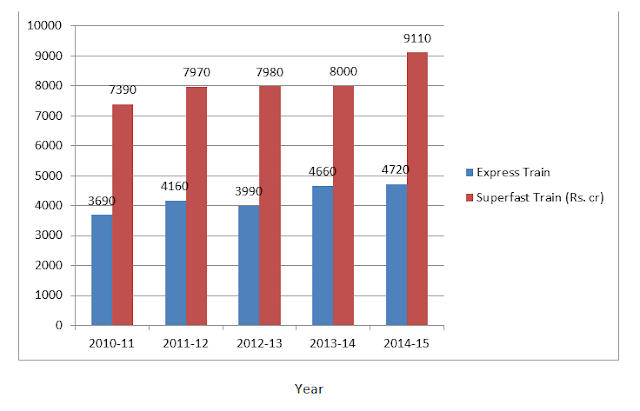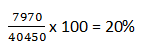## Monday, 20 February 2017

### DI QUIZ3:BASED ON NEW PATTERN

Directions (1-5): Study the following bar graphs to answer the questions given below:
Income of railways from the Super fast trains and Express trainsTotal Expenditure of the Railway on both (Super fast trains and Express trains ) [Profit = Income - Expenditure]1. In which of the following years is the percentage increase/ decrease in the percentage increase/decrease in the total income of the Railways the maximum in comparison to its pevious year?
1. 2012 - 13
2. 2014 -15
3. 2011-12
4. 2013-14
5. Both 1) and 3)

2. In which of the following years is the profit of hte Railways the maximum?
1. 2011-12
2. 2012-13
3. 2013-14
4. Other than the given options
5. 2010- 11

3. In hoe many years is the income from Express trains less than the average income the Express trains in all the given years together?
1. 3
2. 1
3. None
4. Other than the given options
5. 2

4. What is the approxiamate percentage income from Super fast train in 2011-12 in comparison to the total income from Super fast trains for all the given years?
1. Other than the given options
2. 24%
3. 28%
4. 20%
5. 29%

5. The total expenditure of the Railways on both the trains is approximately what percent of the total income of the Railway from both the trains for all the given years together?
1. 83.7%
2. 81.6%
3. Other than the given options
4. 78.9%
5. 86.7%

1. 32. 2;
Profits in (Rs. Crore):
2010 -11 = 1370, 2011-12 = 2270, 2012-13 = 3010
2013-14 = 2060,2014 - 15 = 1330
Hence, maximum profit is in 2012-13

3. 1;
Average income from the Express trainIn 3 years (i.e 2010-11 , 2011-12, 2012-13) income from the Express trains is less than the average income from Express trains.

4. 4;
Required percentage =5. 1;
Required percentage =